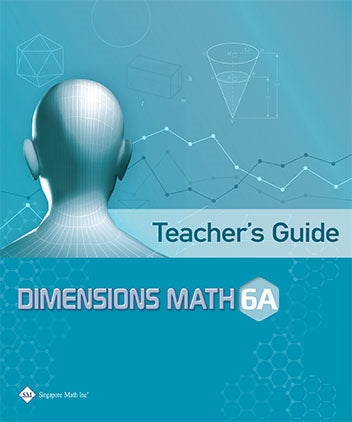# Singapore Math Dimensions Math Teacher's Guide 6A

Dimensions Math® Textbook 6A

Chapter 1: Whole Numbers

1.1 Order of Operations

A. Expressions and Equations
B. Exponents
C. Order of Operations without Parentheses
D. Order of Operations with Parentheses

1.2 Factors and Multiples

A. Factors
B. Multiples

1.3 Multiplication

A. Properties of Multiplication
B. Mental Multiplication

1.4 Division

A. Properties of Division
B. Mental Division

In A Nutshell
Problem Solving Corner

Chapter 2: Fractions

2.1 Multiplication of Fractions

A. Multiplication of a Proper Fraction by a Whole Number
B. Multiplication of a Proper Fraction by a Fraction
C. Multiplication of an Improper Fraction or a Mixed Number by a Whole Number
D. Multiplication of an Improper Fraction or a Mixed Number by a Fraction

2.2 Division of Fractions

A. Division of a Whole Number by a Fraction
B. Division of a Fraction by a Whole Number
C. Division of a Fraction by a Fraction

In A Nutshell
Problem Solving Corner

Chapter 3: Decimals

3.1 Addition and Subtraction of Decimals

A. Structure of Decimals

3.2 Multiplication of Decimals

A. Decimal Number System
B. Multiplier and Product
C. Multiplying Decimals

3.3 Division of Decimals

A. Divisor and Quotient
B. Dividing Decimals

3.4 Metric Measurements and Decimals

In A Nutshell
Problem Solving Corner

Chapter 4: Negative Numbers

4.1 Positive and Negative Numbers
4.2 Comparing Positive and Negative Numbers

A. The Number Line
B. Absolute Value

In A Nutshell

Chapter 5: Ratios

5.1 Ratios and Equivalent Ratios

A. Finding Ratio
B. Equivalent Ratios

5.2 Ratios and Fractions

In A Nutshell
Problem Solving Corner

Chapter 6: Rate

6.1 Average and Rate
6.2 Unit Rate
6.3 Speed

In A Nutshell
Problem Solving Corner

Chapter 7: Percent

7.1 Meaning of Percent
7.2 Percentage of a Quantity

In A Nutshell
Problem Solving Corner

Dimensions Math® G6-8 Textbooks are designed for middle school students. Developed in collaboration between Star Publishing and Singapore Math Inc®, this series follows the Singapore Mathematics Framework and covers the topics in the Common Core State Standards.

The emphasis of this series is on empowering the students to learn mathematics effectively and independently. Depending on the topics covered, different approaches are adopted for the presentation of concepts to facilitate easy understanding by students to internalize concepts and instill in them an interest to explore the topics further.

Each book includes appropriate examples, class activities and diagrams to understand the concepts and apply them. IT skills and values are incorporated as appropriate.

Some features of this series include the following:

1. Class activities which allow the student to learn mathematics through discovery. Some of the class activities make use of Geometer’s Sketchpad (available at keypress.com).
2. Worked examples are followed by a similar question (Try It!) so that students can check if they have understood the concepts presented earlier.
3. An exercise for each lesson including questions in the following sequence:
• Basic Practice (simple questions involving a direct application of the concepts)
• Further Practice (more challenging questions on direct application)
• Maths@Work (questions that apply mathematical concepts to real-life situations)
• Brainworks (questions involving higher order thinking or an open-ended approach to problems)
4. Each chapter is followed by a review exercise; the Extend Your Learning Curve activity encourages students to explore mathematical concepts further or apply mathematics in real-life situations, with some questions requiring sentence or paragraph answers to encourage students to reflect on their learning experiences.
5. The right margins contain remarks on important information, concepts or definitions covered earlier related to the current material to help students recall them, discussion questions, interesting puzzles or facts that are related to mathematics, and some website references.

The answer key at the back of the book provides answers to the Try It! and the problems in the exercises for the Basic PracticeFurther Practice, and Maths@Work questions. It does not include answers to the class activities or the Extend Your Learning Curve activities.